## MP Board Class 6th Maths Solutions Chapter 14 Practical Geometry Ex 14.5

Question 1.
Draw $$\overline{A B}$$ of length 7.3 cm and find its axis of symmetry.
Solution:
Axis of symmetry of line segment $$\overline{A B}$$ will be the perpendicular bisector of $$\overline{A B}$$. So, draw the perpendicular bisector of AB.
Steps of construction:(i) Draw $$\overline{A B}$$ = 7.3 cm.
(ii) With A as centre and radius more than half of AB, draw two arcs, one on each side of AB.
(iii) With B as a centre and the same radius as in step (ii), draw arcs cutting the arcs drawn in the previous step at C and D.
(iv) Join CD. Then CD is the axis of symmetry of the line segment AB.

Question 2.
Draw a line segment of length 9.5 cm and construct its perpendicular bisector.
Solution:
Steps of construction :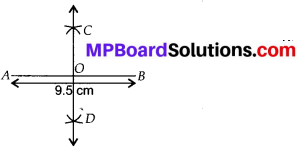(i) Draw $$\overline{A B}$$ = 9.5 cm.
(ii) With A as centre and radius more than half of AB, draw two arcs one on each side of AB.
(iii) With B as a centre and the same radius as in step (ii), draw arcs cutting the arcs drawn in the previous step at C and D.
(iv) Join CD. Then CD is the perpendicular bisector of $$\overline{A B}$$.

Question 3.
Draw the perpendicular bisector of $$\overline{X Y}$$ whose length is 10.3 cm.
(a) Take any point P on the bisector drawn. Examine whether PX = PY.
(b) If M is the mid point of $$\overline{X Y}$$, what can you say about the lengths MX and XY?
Solution:
Steps of construction :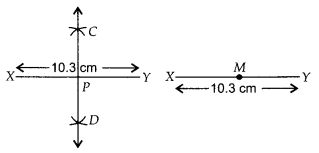(i) Draw $$\overline{X Y}$$ = 10.3 cm.
(ii) With X as centre and radius more than half of XY, draw two arcs one on each side of XY.
(iii) With Y as centre and the same radius as in step (ii), draw two arcs cutting the arcs drawn in the previous step at C and D.
(iv) Join CD. Then CD is the required perpendicular bisector of $$\overline{X Y}$$.
Now
(a) Take any point P on the bisector drawn. With the help of divider we can check that $$\overline{P X}=\overline{P Y}$$ if P is the point of intersection of XY and CD.
(b) If M is the mid-point of $$\overline{X Y}$$, then $$\overline{M X}=\frac{1}{2} \overline{X Y}$$Question 4.
Draw a line segment of length 12.8 cm. Using compasses, divide it into four equal parts. Verify by actual measurement.
Solution:
Steps of construction: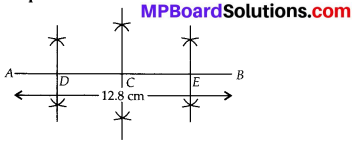(i) Draw AB = 12.8 cm.
(ii) Draw the perpendicular bisector of $$\overline{A B}$$ which cuts it at C. Thus, C is the mid-point of $$\overline{A B}$$.
(iii) Draw the perpendicular bisector of $$\overline{A C}$$ which cuts it at D. Thus D is the mid-point of AC.
(iv) Again, draw the perpendicular bisector of $$\overline{C B}$$ which cuts it at E. Thus, E is the mid-point of $$\overline{C B}$$.
(v) Now, point C, D and E divide $$\overline{A B}$$ in four equal parts.
(vi) By actual measurement, we find that $$\overline{A D}=\overline{D C}=\overline{C E}=\overline{E B}$$ = 3.2 cm

Question 5.
With $$\overline{P Q}$$ of length 6.1 cm as diameter, draw a circle.
Solution:
Steps of construction :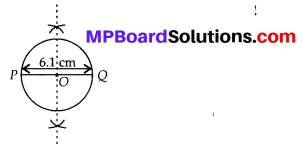(i) Draw a line segment $$\overline{P Q}$$ = 6.1 cm.
(ii) Draw the perpendicular bisector of PQ which cuts, it at O. Thus O is the mid-point of $$\overline{P Q}$$.
(iii) Taking O as centre and OP or OQ as radius draw a circle where $$\overline{P Q}$$ is the diameter.

Question 6.
Draw a circle with centre C and radius 3.4 cm. Draw any chord $$\overline{A B}$$. Construct the perpendicular bisector of $$\overline{A B}$$ and examine if it passes through C.
Solution:
Steps of construction: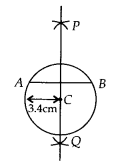(i) Draw a circle with centre C and radius 3.4 cm.
(ii) Draw any chord $$\overline{A B}$$.
(iii) With A as center and radius more than half of $$\overline{A B}$$, draw two arcs one on each side of AB.
(iv) With B as a centre and the radius same as in step (iii), draw two arcs cutting the arcs drawn in the previous step at P and Q.
(v) Join PQ. Then PQ is the perpendicular bisector of $$\overline{A B}$$.
(vi) This perpendicular bisector of $$\overline{A B}$$ passes through the centre C of the circle.Question 7.
Repeat Question 6, if $$\overline{A B}$$ happens to be a diameter.
Solution:
Steps of construction :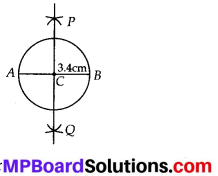(i) Draw a circle with centre C and radius 3.4 cm.
(ii) Draw its diameter AB.
(iii) With A as center and radius more than half of AB, draw two arcs one on each side of AB.
(iv) With B as a centre and the radius same as in step (iii), draw two arcs cutting the arcs drawn in the previous step at P and Q.
(v) Join PQ. Then PQ is the perpendicular bisector of $$\overline{A B}$$.
(vi) We observe that this perpendicular bisector of $$\overline{A B}$$ intersect it at the centre C of the circle.

Question 8.
Draw a circle of radius 4 cm. Draw any two of its chords. Construct the perpendicular bisectors of these chords. Where do they meet?
Solution:
Steps of construction :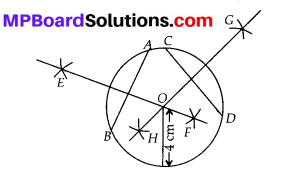(i) Draw the circle with centre O and radius 4 cm.
(ii) Draw any two chords AB and CD in this, circle.
(iii) With A as center and radius more than half AB, draw two arcs one on each side of AB.
(iv) With B as centre and radius same as in step (ii), draw two arcs cutting the arcs drawn in previous step at E and F.
(v) Join EF. Thus EF is the perpendicular bisector of chord AB.
(vi) Similarly, draw GH the perpendicular bisector of chord CD.
(vii) These two perpendicular bisectors meet at O, the centre of the circle.

Question 9.
Draw any angle with vertex O. Take a point A on one’ of its arms and B on another such that OA = OB. Draw the perpendicular bisectors of $$\overline{O A}$$ and $$\overline{O B}$$. Let them meet at P. Is PA = PB?
Solution:
Steps of construction :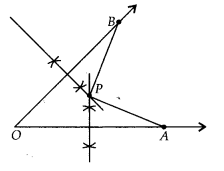(i) Draw any angle with vertex O.
(ii) Take a point A on one of its arms and B on another such that OA = OB.
(iii) Draw perpendicular bisector of OA and OB.
(iv) Let they meet at P. Join PA and PB.
(v) With the help of divider, we check that PA = PB.